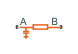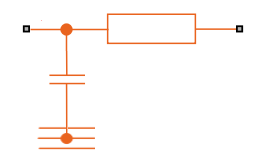# Cauer Thermal Model

Heat transfer through multiple layers of a semiconductor module

• Library:
• Simscape / Electrical / Passive / Thermal

•## Description

The Cauer Thermal Model block represents heat transfer through multiple layers of a semiconductor module. A Cauer Thermal Model contains multiple Cauer Thermal Model Element components. The figure shows an equivalent circuit for a Cauer Thermal Model Element.The multiple layers of a Cauer thermal model include chip, solder, substrate, solder, and base. Other terms that describe a Cauer thermal model are:

• Continued fraction circuit

• T model

This figure shows an equivalent circuit for a Cauer Thermal Model.The temperature at R is equal to the absolute zero temperature.

### Equations

The defining equations for each Cauer thermal model element are

`${C}_{thermal}=\frac{\tau }{{R}_{thermal}},$`
`${Q}_{AB}=\frac{{T}_{AB}}{{R}_{thermal}},$`

and

`${Q}_{AR}={C}_{thermal}\frac{d{T}_{AR}}{dt},$`

where:

• Cthermal is the thermal capacity.

• τ is the thermal time constant.

• Rthermal is the thermal resistance.

• QAB is the heat flow through the material.

• TAB is the temperature difference between the material layers.

• QAR is the heat flow through the thermal capacity.

• TAR is the temperature drop across the thermal capacity.

### Parameterize Cauer Model from Foster Coefficients

The datasheets of semiconductor devices often use Foster coefficients to specify the thermal model. However, Cauer thermal models are more useful as you can extend them with additional thermal components such as heatsinks and radiation or convection elements.

To parameterize the Cauer Thermal Model block from Foster thermal model data, select the Parameterize Cauer model using Foster coefficient data parameter.

### Variables

To set the priority and initial target values for the block variables prior to simulation, use the Initial Targets section in the block dialog box or Property Inspector. For more information, see Set Priority and Initial Target for Block Variables.

Nominal values provide a way to specify the expected magnitude of a variable in a model. Using system scaling based on nominal values increases the simulation robustness. Nominal values can come from different sources, one of which is the Nominal Values section in the block dialog box or Property Inspector. For more information, see System Scaling by Nominal Values.

To specify the initial temperatures of each thermal capacity, set the value of the Vector of thermal mass temperatures variable. The length of this vector must be equal to the number of Cauer thermal model elements. It corresponds to the temperature drop across each thermal capacity in the model.

## Ports

### Conserving

expand all

Thermal conserving port associated with the first surface of the individual layer of the semiconductor.

Thermal conserving port associated with the second surface of the last layer of the semiconductor.

## Parameters

expand all

Whether to parameterize the Cauer model by using coefficient data from a Foster model.

Vector of thermal resistances.

#### Dependencies

To enable this parameter, clear the Parameterize Cauer model using Foster coefficient data parameter.

Vector of thermal time constants.

#### Dependencies

To enable this parameter, clear the Parameterize Cauer model using Foster coefficient data parameter.

Vector of thermal resistances parameterized by using data from a Foster model.

#### Dependencies

To enable this parameter, select the Parameterize Cauer model using Foster coefficient data parameter.

Vector of thermal time constants parameterized by using data from a Foster model.

#### Dependencies

To enable this parameter, select the Parameterize Cauer model using Foster coefficient data parameter.

 Schütze, T. AN2008-03: Thermal equivalent circuit models. Application Note. V1.0. Germany: Infineon Technologies AG, 2008.

 T. G. Subhash Joshi and V. John, Combined transient thermal impedance estimation for pulse-power applications. 2017 National Power Electronics Conference (NPEC), 2017, pp. 42-47, doi: 10.1109/NPEC.2017.8310432.